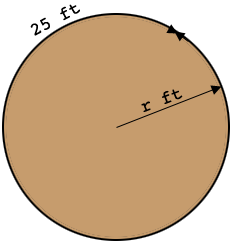SEARCH HOMEMath Central Quandaries & QueriesQuestion from Jackie: Filling a hole 25ft round 3ft deep how much dirt is needed?Hi Jackie,

The volume of the hole is the area of the circular top times the depth. I remember that the area $A$ of a circle is given by $A = \pi \; r^2$ where $r$ is the radius. But I don't think you have the radius but you have the distance around, what I would call the circumference of the circle. This is my diagram.The circumference $C$ of a circle of radius $r$ is given by $C = 2 \; \pi \; r.$

If you solve this equation for $r$ and substitute into the equation for the area you get

$A = \frac{C^2}{4 \; \pi}.$

When I calculated this for $C = 25$ ft I got $A = 1.99$ square feet. (you should check my arithmetic.) The volume of your hole is hence $1.99 \times 3$ cubic feet.

I hope this helps,
PennyMath Central is supported by the University of Regina and The Pacific Institute for the Mathematical Sciences.